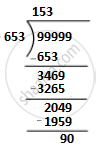# Divide the largest 5 digit number by 653. Check your answer by the division algorithm. - Mathematics

Fill in the Blanks

Divide the largest 5 digit number by 653. Check your answer by the division algorithm.

#### Solution

Largest five-digit number = 99999Dividend = 99999, Divisor = 653, Quotient = 153 and Remainder = 90
Check: Divisor ×Quotient + Remainder
= 653 × 153 + 90
= 99909 + 90
= 99999
= Dividend
∴ Dividend = Divisor × Quotient + Remainder

Is there an error in this question or solution?

#### APPEARS IN

RS Aggarwal Class 6 Mathematics
Chapter 3 Whole Numbers
Exercise 3E | Q 11 | Page 56